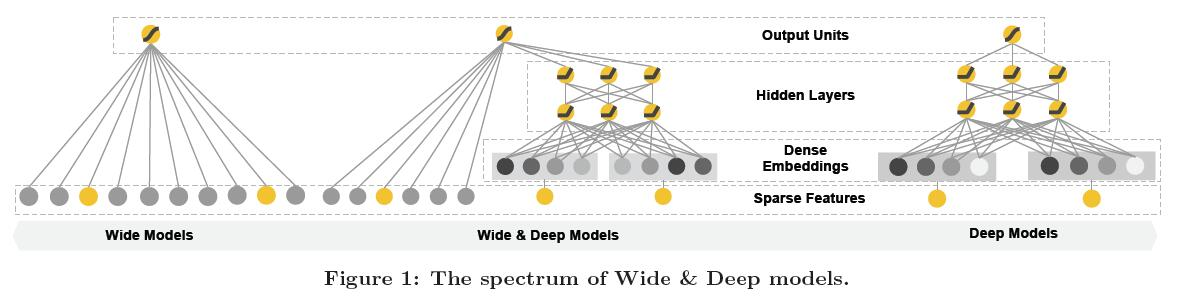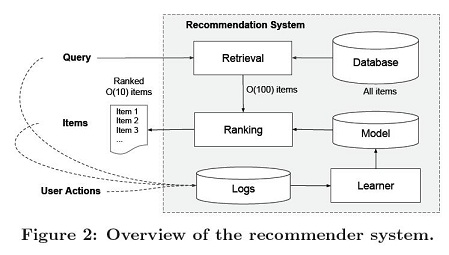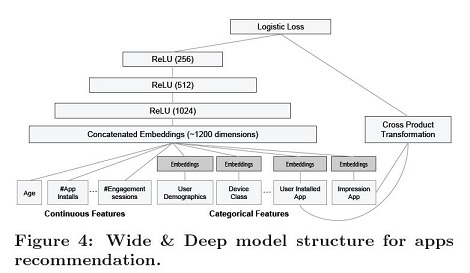# 1. 概述

• 记忆（memorization）即从历史数据中发现item或者特征之间的相关性。
• 泛化（generalization）即相关性的传递，发现在历史数据中很少或者没有出现的新的特征组合。

# 2. Wide & Deep模型

## 2.1. Wide & Deep模型结构

Wide & Deep模型的结构如下图所示：## 2.2. Wide模型

Wide模型如上图中的左侧的图所示，实际上，Wide模型就是一个广义线性模型：

y = w T x + b y=\mathbf{w}^T\mathbf{x}+b

## 2.3. Deep模型

Deep模型如上图中的右侧的图所示，实际上，Deep模型是一个前馈神经网络。深度神经网络模型通常需要的输入是连续的稠密特征，对于稀疏，高维的类别特征，通常首先将其转换为低维的向量，这个过程也成为embedding。

a ( l + 1 ) = f ( W ( l ) a ( l ) + b ( l ) ) a^{\left ( l+1 \right )}=f\left ( W^{\left ( l \right )}a^{(l)}+b^{\left ( l \right )} \right )

## 2.4. Wide & Deep模型的联合训练（joint training）

P ( Y = 1 ∣ x ) = σ ( w w i d e T [ x , ϕ ( x ) ] + w d e e p T a ( l f ) + b ) P\left ( Y=1\mid \mathbf{x} \right )=\sigma \left ( \mathbf{w}_{wide}^T\left [ \mathbf{x},\phi \left ( \mathbf{x} \right ) \right ] + \mathbf{w}_{deep}^Ta^{\left ( l_f \right )}+b \right )

• Wide模型：FTRL
• Deep模型：AdaGrad

# 3. apps的推荐系统

## 3.1. 推荐系统## 3.2. apps推荐的特征# 参考文献

1. Cheng H T, Koc L, Harmsen J, et al. Wide & Deep Learning for Recommender Systems[J]. 2016:7-10.
2. wide & Deep 和 Deep & Cross 及tensorflow实现
3. Wide & Deep 的官方实现
4. 深度学习在 CTR 中应用
5. 《Wide & Deep Learning for Recommender Systems 》笔记
6. 深度学习在美团点评推荐平台排序中的运用
7. tensorflow线性模型以及Wide deep learning
07-2407-032万+
12-134252
03-142万+
04-1913万+
07-062642
04-213381
10-124404
01-104万+
03-281642
11-11133
08-24
06-131273
03-177万+
09-16710
09-17304
09-191559
05-14387
02-161531
©️2020 CSDN 皮肤主题: 编程工作室 设计师:CSDN官方博客